# 深度解析射击游戏的「打击感」！Cocos Creator 实现超爽枪弹射击效果COCOS |376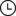2021-12-30 14:04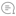0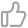00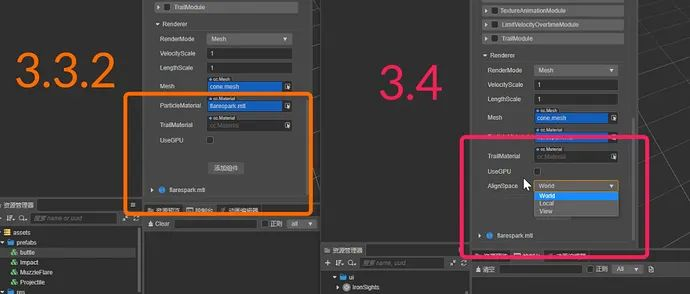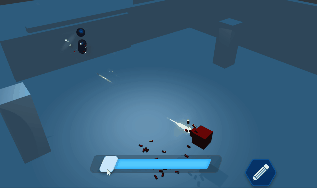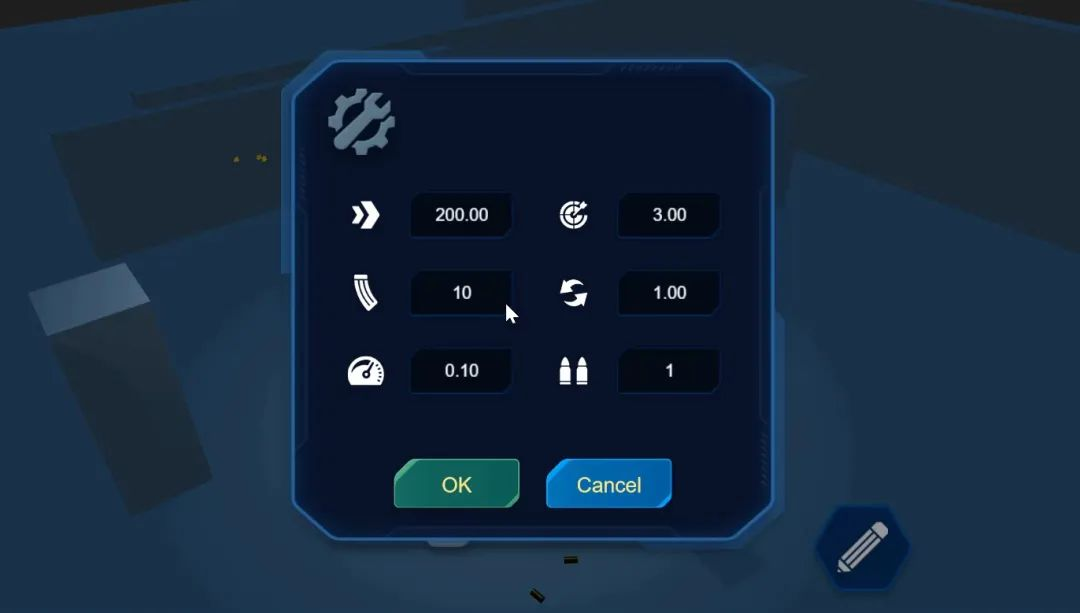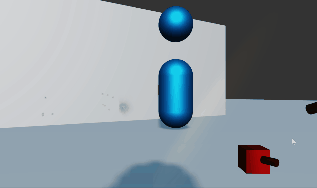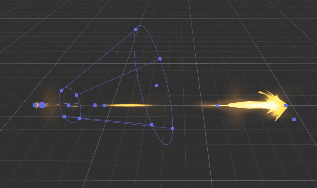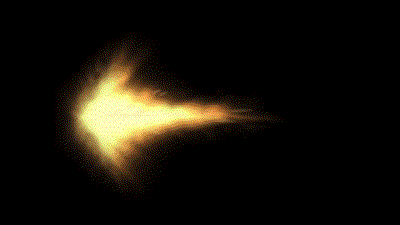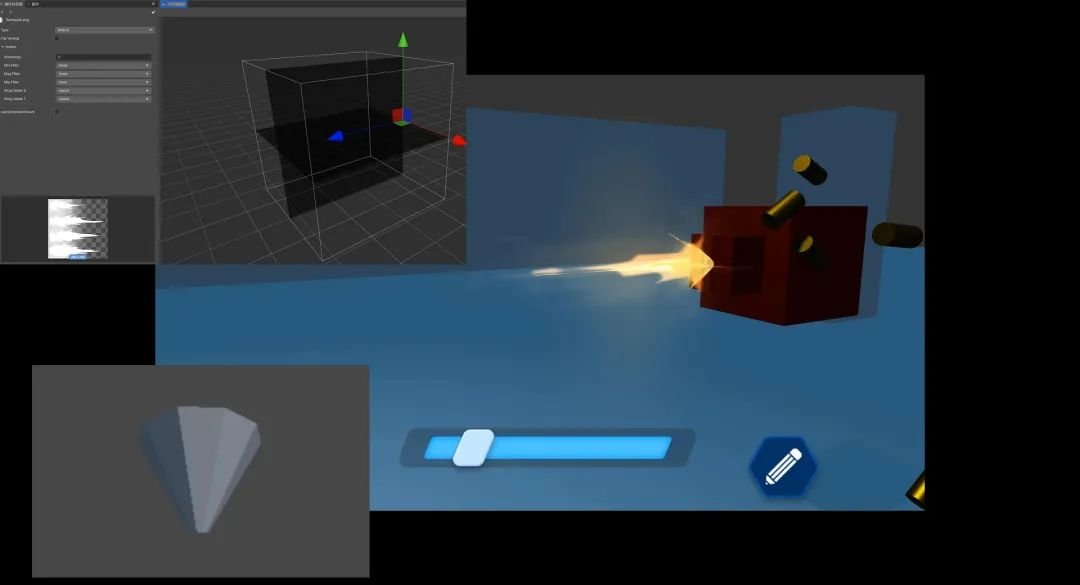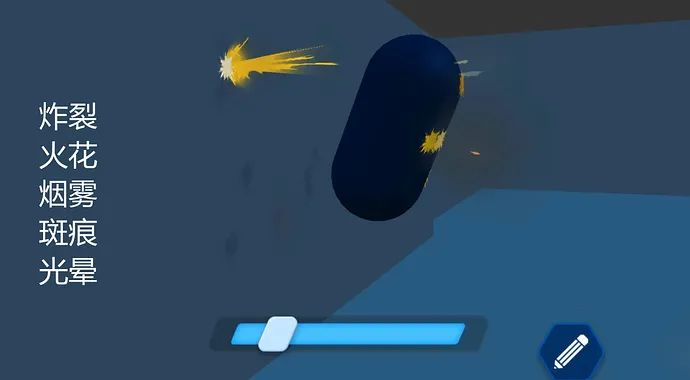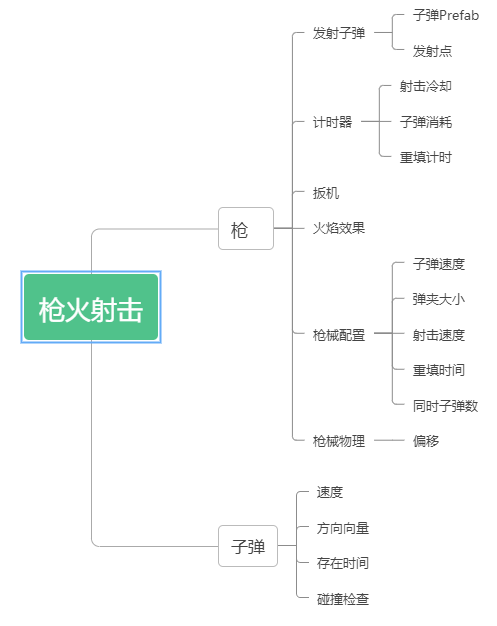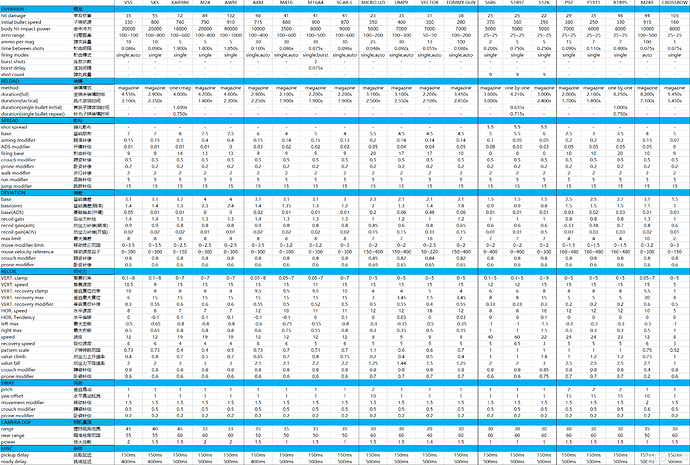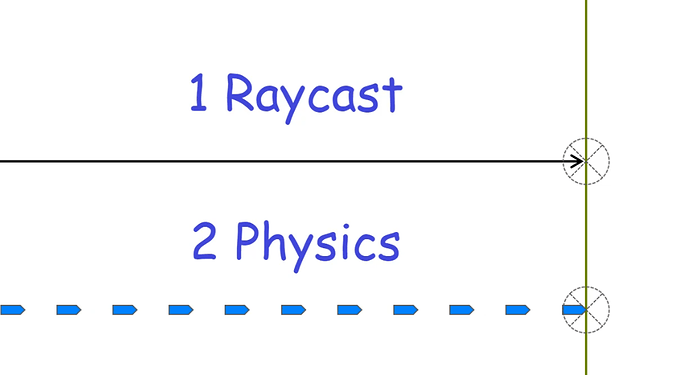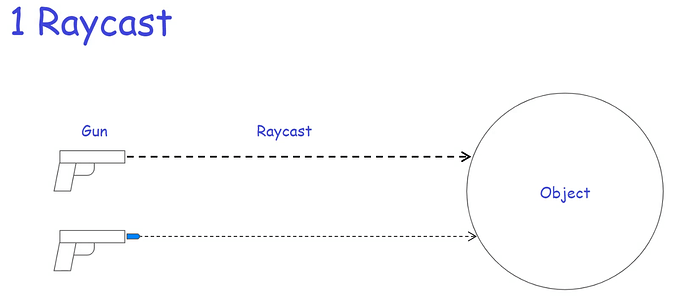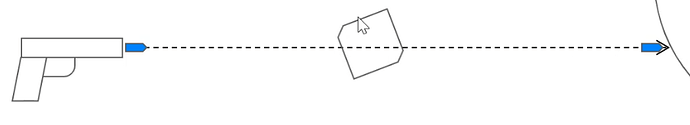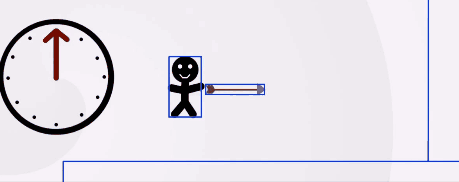BulletSc.ts 的代码：

`import { _decorator, Component,  Vec3, v3, geometry, physics, RigidBody, game } from 'cc';import { AutoRecycleSc } from './AutoRecycleSc';import { ImpactHelperSc } from './ImpactHelperSc';const { ccclass, property } = _decorator;@ccclass('BulletSc')export class BulletSc extends Component {    private _speed: number = 200;    public get speed(): number {        return this._speed;    }    public set speed(v: number) {        this._speed = v;        if (this._vector) {            this._vector = this._vector.normalize().multiplyScalar(this.speed);        }    }    private _vector: Vec3 = null;    setVector(v: Vec3) {        this._vector = v.clone().multiplyScalar(this.speed);        this.node.forward = v;        BulletSc.preCheck(this, this.speed / 60);    }    private _vec3 = v3();    update(deltaTime: number) {        if (this._vector) {            Vec3.multiplyScalar(this._vec3, this._vector, deltaTime);            this.node.position = this.node.position.add(this._vec3);            BulletSc.preCheck(this, this._vec3.length());        }    }    private static preCheck(b: BulletSc, len: number) {        const p = b.node.worldPosition;        const v = b._vector;        const ray = geometry.Ray.create(p.x, p.y, p.z, v.x, v.y, v.z);        const phy = physics.PhysicsSystem.instance;        if (phy.raycast(ray, 0xffffff, len)) {            if (phy.raycastResults.length > 0) {                let result = phy.raycastResults;                    game.emit(ImpactHelperSc.AddImpactEvent,b,result);                if (result.collider.getComponent(RigidBody)) {                    result.collider.getComponent(RigidBody).applyForce(b._vector, result.hitPoint);                }                if (b.getComponent(AutoRecycleSc))                    b.getComponent(AutoRecycleSc).recycle();            }        }    }}`

`import { _decorator, Component, Node } from 'cc';const { ccclass, property } = _decorator;@ccclass('AutoRecycleSc')export class AutoRecycleSc extends Component {    @property    deltaTime = 5;    update (dt: number) {        this.deltaTime -= dt;        if(this.deltaTime <=0){            this.recycle();        }    }    recycle(){        this.deltaTime = 1000;        this.node.destroy();    }}`

`@ccclass("gun_overview")export class GunOverView {    @property    bulletSpeed = 200;    @property    ammoPerMag: number = 10;    @property    timeBetweenShots: number = 0.3;    @property    timeReload: number = 1;    @property    meanwhile: number = 1;    @property    speadValue: number = 1;}`

`isShotting = true;private vec3: Vec3 = v3();private timer: Timer = new Timer();// other classclass Timer {    shot: number = 0;    ammo: number = 0;    reload: number = 0;}`

`createBullet() {// to create bullet}`

`// ... Part@property(GunOverView)gunOverview: GunOverView = new GunOverView();@property(Node)fireEffect: Node = null;@property(Prefab)bullet: Prefab = null;@property(Node)muzzleNode: Node = null;// ... PartcreateBullet() {         this.vec3 = this.muzzleNode.forward.clone();    const b = instantiate(this.bullet);    director.getScene().addChild(b);    b.setWorldPosition(this.muzzleNode.worldPosition);    b.setWorldRotation(this.muzzleNode.worldRotation);    b.getComponent(BulletSc).speed = this.gunOverview.bulletSpeed;    let rot = this._quat;    const speadValue = this.gunOverview.speadValue;    Quat.fromEuler(rot, (Math.random() * 2 - 1) * speadValue,        (Math.random() * 2 - 1) * speadValue, (Math.random() * 2 - 1) * speadValue);    Vec3.transformQuat(this.vec3,this.vec3.normalize(),rot);    b.forward = this.vec3;    b.getComponent(BulletSc).setVector(b.forward);    b.worldScale = this.muzzleNode.worldScale;}// ... Part`

`update(dt:number){    if(!this.isShotting)return;    if(this.timer.ammo > 0){        this.timer.shot += dt;        if(this.timer.shot >= this.gunOverview.timeBetweenShots){            this.timer.shot = 0;            this.shot();            this.timer.ammo -= 1;            this.timer.reload = 0;            if(this.timer.ammo <=0){                //重填            }        }    }else{        if(this.timer.reload >= this.gunOverview.timeReload){            this.timer.ammo = this.gunOverview.ammoPerMag;            this.timer.reload = 0;        }        this.timer.reload += dt;    }}resetState(){    this.timer.shot = this.timer.ammo = this.timer.reload = 0;}`

ParticleEffectHelper.ts

``import { ParticleSystem,Node } from "cc";export module ParticleEffectHelper{    export function Play(node:Node){        const arr = node.getComponentsInChildren(ParticleSystem);        for(let a of arr){            a.stop();            a.play();        }    }}``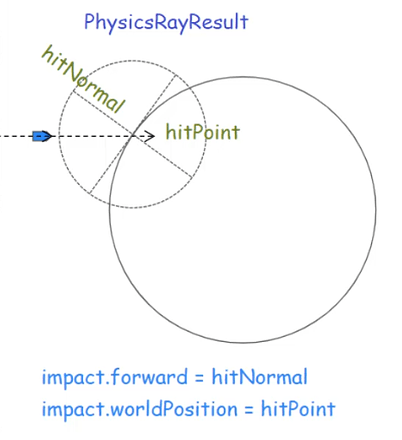ImpactHelperSc.ts

``import { _decorator, Component, Node, Prefab, game, PhysicsRayResult, instantiate } from 'cc';import { BulletSc } from './BulletSc';const { ccclass, property } = _decorator; @ccclass('ImpactHelperSc')export class ImpactHelperSc extends Component {    public static AddImpactEvent:string = "AddImpactEvent";    @property(Prefab)    impact1:Prefab = null;    start () {        game.on(ImpactHelperSc.AddImpactEvent,<any>this.onAddImpactEvent,this);    }    private onAddImpactEvent(b:BulletSc,e:PhysicsRayResult) {        const impact = instantiate(this.impact1);        impact.worldPosition = e.hitPoint.add(e.hitNormal.multiplyScalar(0.01));        impact.forward=e.hitNormal.multiplyScalar(-1);        impact.scale = b.node.scale;        impact.setParent(e.collider.node,true);            }}``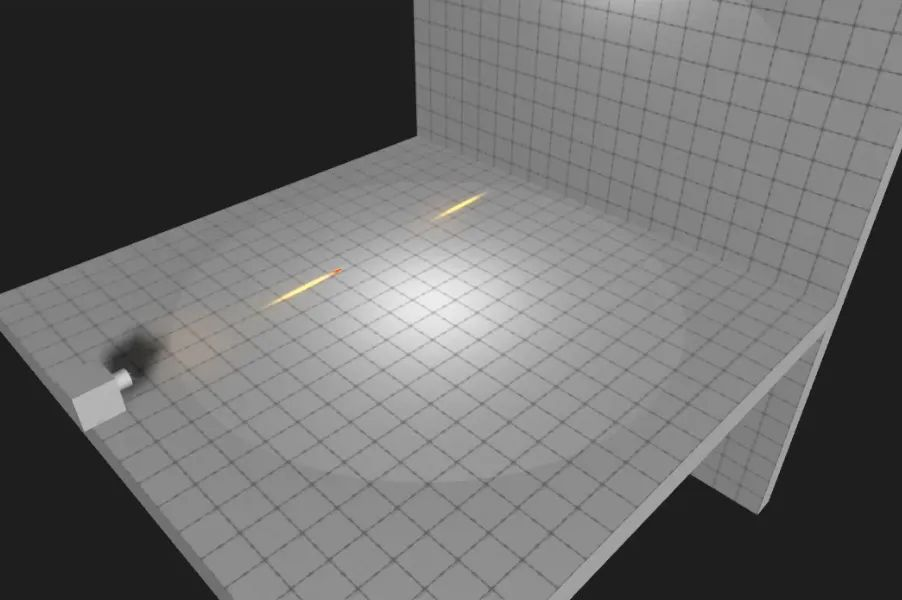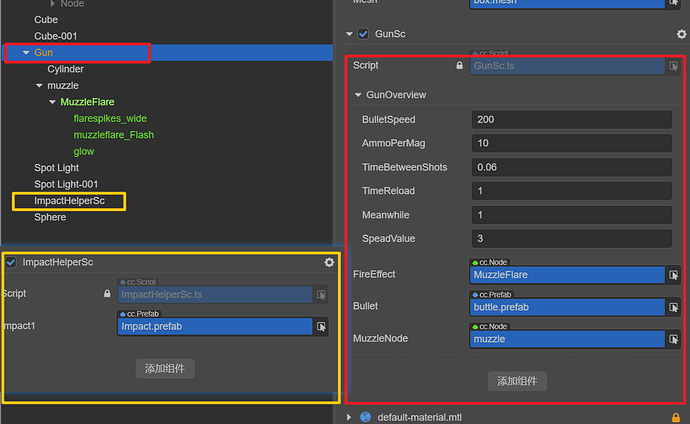https://www.bilibili.com/video/BV15u411S7Mz

https://store.cocos.com/app/detail/3473

Demo 地址：

https://forum.cocos.org/t/topic/127488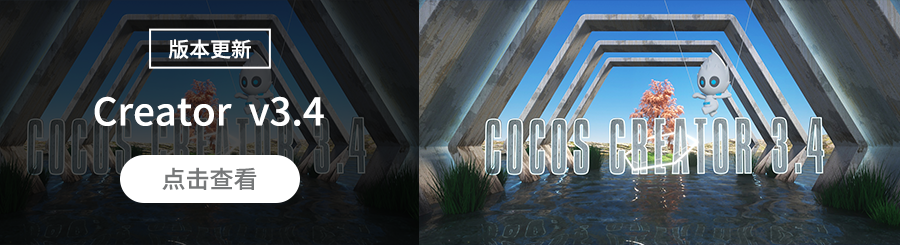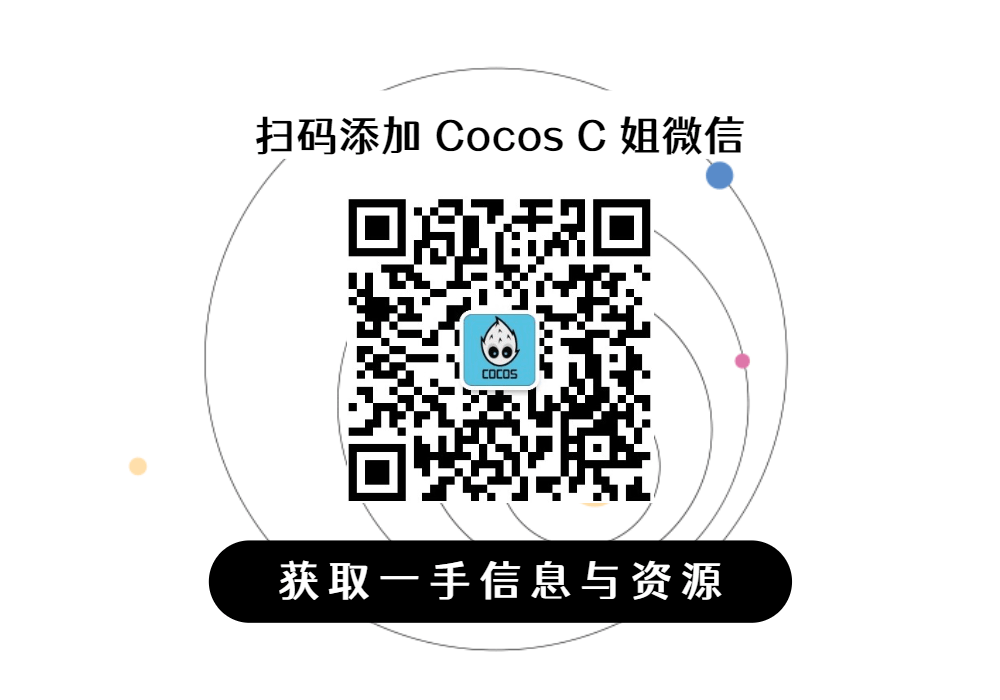00

暂无评论~~
Ctrl+Enter